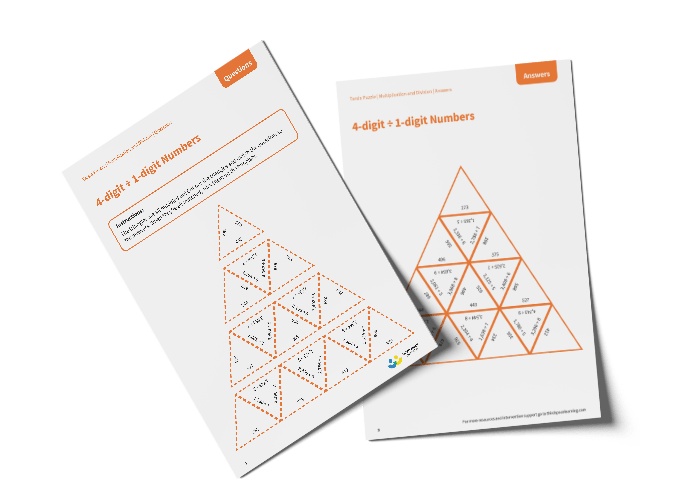# Tarsia Puzzle 4-digit Divide by 1-digit Numbers (Year 5)

The triangles in the tarsia puzzle have all been mixed up. In this 4-digit divide by 1-digit numbers tarsia puzzle, pupils will need to match the division question to the correct answer to create one large triangle.

This Year 5, 4-digit divide by 1-digit numbers tarsia puzzle worksheet contains:

• One question sheet
• One template sheet

Year 5

Division